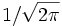# Fourier transformationThis article/section deals with mathematical concepts appropriate for late high school or early college.

The Fourier transformation (often called the Fourier transform or Fourier integral) is an invertible integral transformation that decomposes a square integrable, piecewise continuous functions on a topological group into a linear combination of basis elements. It can be thought of as the ultimate extension of the Fourier series, in which the interval of periodicity becomes infinitely long and the "coefficients" infinitely close together, becoming a function instead of an infinite series.

Often, functions which are difficult to analyze in one topological group become much easier to analyze when transformed to another topological group.

The formulas usually favored by mathematicians are the "normalized" form. Given a functiondefined on the entire real line, its Fourier transformis given by:The inverse transform, that recovers the original function, is:The constants in front of the integrals are arbitrary, so long as their product is. In order to make the forward and inverse transforms as similar as possible, an oft-used convention is to set them both equal to. The Fourier transform of a functionis often denoted with a hat:## Discrete Fourier transformation

Discrete Fourier transformations are defined on discrete topological groups, and the integral is replaced by summation.